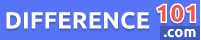Irrational Number

An irrational number simply can’t be expressed as a rational number. In other words, it can’t be expressed as a fraction of two integers (x) and (y). An irrational number’s decimal expansion is neither recurring nor finite – it just goes on forever. Common irrational numbers are π (3.14…) and e (2.718…). Surds are another type of irrational number – they are non-perfect cubes or squares that can’t be reduced further to remove a cube root or square root. For example, √2 is an irrational number because it cannot be expressed as a rational number. However, √4 is a rational number because it can be expressed as 2/2 (two over two)WordPress database error: [Unknown column 'post_id' in 'where clause']
`SELECT * FROM wp_qss WHERE post_id = 7434`

WordPress database error: [Unknown column 'post_id' in 'where clause']
`SELECT * FROM wp_qss WHERE post_id = 7434`

WordPress database error: [Unknown column 'post_id' in 'where clause']
`SELECT * FROM wp_qss WHERE post_id = 7434`

WordPress database error: [Unknown column 'post_id' in 'where clause']
`SELECT * FROM wp_qss WHERE post_id = 7434`